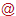Leibniz Institute for Plasma Science and Technology
Felix-Hausdorff-Str. 2
17489 Greifswald
GERMANY

https://www.inp-greifswald.de/en/
welcomeinp-greifswald [punkt] de

The Leibniz Institute for Plasma Science and Technology (INP) is the largest non-university institute in the field of low temperature plasmas, their basics and technical applications in Europe. The institute carries out research and development from idea to prototype. The topics focus on the needs of the market. At present, plasmas for materials and energy as well as for environment and health are the focus of interest.

## Introduction and verification of FEDM, an open-source FEniCS-based discharge modelling code - dataset

The dataset contains the data presented in the paper introducing the FEDM (Finite Element Discharge Modelling) code. The FEDM code was developed using the open-source computing platform FEniCS (https://fenicsproject.org). Building on FEniCS, the FEDM code utilises the finite element method to solve partial differential equations. It extends FEniCS with features that allow the automated implementation and numerical solution of fully-coupled fluid-Poisson models, including an arbitrary number of particle balance equations. The code is verified using the method of exact solutions and benchmarking. The physically based examples of a time-of-flight experiment, a positive streamer discharge in atmospheric-pressure air and a low-pressure glow discharge in argon are used as rigorous test cases for the developed modelling code and to illustrate its capabilities. The performance of the code is compared to the commercial software package COMSOL Multiphysics®, and a comparable parallel speed-up is obtained. It is shown that the iterative solver implemented by FEDM performs particularly well on high-performance compute clusters.

FieldValue
Group
Authors
Release Date
2023-04-04
Identifier
Permanent Identifier (DOI)
Permanent Identifier (URI)
Is supplementing
Plasma Source Name
Plasma Source Application
Plasma Source Specification
Plasma Source Properties

The first case considers a time-of-flight experiment at atmospheric pressure of 760 Torr and a constant gas temperature of 300 K. The configuration consists of two plane-parallel electrodes with a gap distance of 1 mm and a radius of 0.5 mm. The constant field of 3.55 MV/m was assumed in order to model the spatiotemporal evolution of an electron cloud.

The second case considers a positive streamer in atmospheric-pressure air and a gas temperature of 300 K. Two electrodes are set 1.25 cm apart, while their radius is 1.25 cm. The constant voltage of -18.75 kV was applied at the powered electrode, while an additional Gaussian seed of ions was introduced near the anode to enhance the electric field above the breakdown threshold locally.

The third case considers abnormal glow discharge in argon at low pressure of 1 Torr. The plane-parallel electrodes with the radius of 1 cm were set 1 cm apart. The exponentially rising voltage with U_0 = -250 V and time constant of 1 ns was applied to the powered electrode.

Plasma Medium Name
Plasma Medium Properties

First case (time-of-flight experiment): synthetic air, gas pressure is 760 Torr, constant gas temperature of 300 K

Second case (streamer benchmark): synthetic air, gas pressure is 760 Torr, constant gas temperature of 300 K

Third case (abnormal glow discharge): pure argon, gas pressure is 1 Torr, constant gas temperature of 300 K

Plasma Diagnostics Name
Plasma Diagnostics Properties

Model: time-dependent fluid-Poisson model in spatially two-domensional (2D) cylindrical geometry;

Computational software: finite element discharge modelling (FEDM) code (https://github.com/INP-PM/FEDM/releases/tag/v1.0.0), powered by FEniCS (https://fenicsproject.org); COMSOL Multiphysics® v. 5.6 (www.comsol.com, COMSOL AB, Stockholm, Sweden) as a benchmark;

Numerical method: finite element method; element type: linear Lagrange elements for particle balance equations and Poisson equation; time-stepping: Backward differentiation formula (BDF), with automatically adapted time-step size;

Solver: fully coupled Newton solver, with direct linear (MUMPS for FEDM, and PARDISO and MUMPS for COMSOL Multiphysics®) and iterative (gmres with hypre preconditioner) solver; nonlinear solver tolerance: 0.0001; time stepping tolerance: varied for different case studies;

Plasma Diagnostics Procedure

The FEDM code verification was performed using the method of exact solutions and benchmarking. In the first study, the time-of-flight experiment is modelled. The calculated electron number density was compared to the analytically derived exact solution, and rigorous code verification was carried out by performing order-of-accuracy studies for the space and time discretisation of the particle balance equation. The modelling of the positive streamer benchmark in the air at atmospheric pressure proposed by Bagheri B et al. 2018 Plasma Sources Sci. Technol. 27 095002 was used for a further, less rigorous verification of the FEDM code. This benchmarking is useful for verifying modelling codes for cases where an analytical solution is unavailable. The modelling of an abnormal glow discharge in argon at low pressure was used to illustrate all FEDM code features and for further benchmarking by comparing the results to the commercial software package COMSOL Multiphysics®. The performance of the code was compared to the commercial software, and the influence of the choice of the linear solver on the code performance was tested by measuring the parallel speed-up.

Language
English